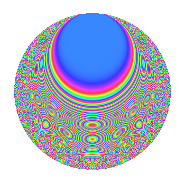Properties

 Label 4021.2.a.bLevel 4021 Weight 2 Character orbit 4021.a Self dual Yes Analytic conductor 32.108 Analytic rank 1 Dimension 151 CM No

Related objects

Newspace parameters

 Level: $$N$$ = $$4021$$ Weight: $$k$$ = $$2$$ Character orbit: $$[\chi]$$ = 4021.a (trivial)

Newform invariants

 Self dual: Yes Analytic conductor: $$32.1078466528$$ Analytic rank: $$1$$ Dimension: $$151$$ Fricke sign: $$1$$ Sato-Tate group: $\mathrm{SU}(2)$

$q$-expansion

The dimension is sufficiently large that we do not compute an algebraic $$q$$-expansion, but we have computed the trace expansion.

 $$\operatorname{Tr}(f)(q) =$$ $$151q$$ $$\mathstrut -\mathstrut 16q^{2}$$ $$\mathstrut -\mathstrut 29q^{3}$$ $$\mathstrut +\mathstrut 120q^{4}$$ $$\mathstrut -\mathstrut 27q^{5}$$ $$\mathstrut -\mathstrut 18q^{6}$$ $$\mathstrut -\mathstrut 18q^{7}$$ $$\mathstrut -\mathstrut 48q^{8}$$ $$\mathstrut +\mathstrut 98q^{9}$$ $$\mathstrut +\mathstrut O(q^{10})$$ $$\operatorname{Tr}(f)(q) =$$ $$151q$$ $$\mathstrut -\mathstrut 16q^{2}$$ $$\mathstrut -\mathstrut 29q^{3}$$ $$\mathstrut +\mathstrut 120q^{4}$$ $$\mathstrut -\mathstrut 27q^{5}$$ $$\mathstrut -\mathstrut 18q^{6}$$ $$\mathstrut -\mathstrut 18q^{7}$$ $$\mathstrut -\mathstrut 48q^{8}$$ $$\mathstrut +\mathstrut 98q^{9}$$ $$\mathstrut -\mathstrut 7q^{10}$$ $$\mathstrut -\mathstrut 126q^{11}$$ $$\mathstrut -\mathstrut 47q^{12}$$ $$\mathstrut -\mathstrut 10q^{13}$$ $$\mathstrut -\mathstrut 58q^{14}$$ $$\mathstrut -\mathstrut 50q^{15}$$ $$\mathstrut +\mathstrut 74q^{16}$$ $$\mathstrut -\mathstrut 39q^{17}$$ $$\mathstrut -\mathstrut 47q^{18}$$ $$\mathstrut -\mathstrut 48q^{19}$$ $$\mathstrut -\mathstrut 64q^{20}$$ $$\mathstrut -\mathstrut 50q^{21}$$ $$\mathstrut -\mathstrut 7q^{22}$$ $$\mathstrut -\mathstrut 91q^{23}$$ $$\mathstrut -\mathstrut 44q^{24}$$ $$\mathstrut +\mathstrut 102q^{25}$$ $$\mathstrut -\mathstrut 94q^{26}$$ $$\mathstrut -\mathstrut 92q^{27}$$ $$\mathstrut -\mathstrut 27q^{28}$$ $$\mathstrut -\mathstrut 79q^{29}$$ $$\mathstrut -\mathstrut 12q^{30}$$ $$\mathstrut -\mathstrut 33q^{31}$$ $$\mathstrut -\mathstrut 102q^{32}$$ $$\mathstrut -\mathstrut 7q^{33}$$ $$\mathstrut -\mathstrut 17q^{34}$$ $$\mathstrut -\mathstrut 183q^{35}$$ $$\mathstrut -\mathstrut 6q^{36}$$ $$\mathstrut -\mathstrut 24q^{37}$$ $$\mathstrut -\mathstrut 77q^{38}$$ $$\mathstrut -\mathstrut 81q^{39}$$ $$\mathstrut -\mathstrut 37q^{40}$$ $$\mathstrut -\mathstrut 62q^{41}$$ $$\mathstrut +\mathstrut 4q^{42}$$ $$\mathstrut -\mathstrut 74q^{43}$$ $$\mathstrut -\mathstrut 209q^{44}$$ $$\mathstrut -\mathstrut 35q^{45}$$ $$\mathstrut -\mathstrut 37q^{46}$$ $$\mathstrut -\mathstrut 127q^{47}$$ $$\mathstrut -\mathstrut 59q^{48}$$ $$\mathstrut +\mathstrut 75q^{49}$$ $$\mathstrut -\mathstrut 79q^{50}$$ $$\mathstrut -\mathstrut 138q^{51}$$ $$\mathstrut -\mathstrut 12q^{52}$$ $$\mathstrut -\mathstrut 104q^{53}$$ $$\mathstrut -\mathstrut 43q^{54}$$ $$\mathstrut -\mathstrut 49q^{55}$$ $$\mathstrut -\mathstrut 167q^{56}$$ $$\mathstrut -\mathstrut 19q^{57}$$ $$\mathstrut -\mathstrut 32q^{58}$$ $$\mathstrut -\mathstrut 207q^{59}$$ $$\mathstrut -\mathstrut 94q^{60}$$ $$\mathstrut -\mathstrut 57q^{61}$$ $$\mathstrut -\mathstrut 69q^{62}$$ $$\mathstrut -\mathstrut 49q^{63}$$ $$\mathstrut -\mathstrut 4q^{64}$$ $$\mathstrut -\mathstrut 78q^{65}$$ $$\mathstrut -\mathstrut 51q^{66}$$ $$\mathstrut -\mathstrut 92q^{67}$$ $$\mathstrut -\mathstrut 80q^{68}$$ $$\mathstrut -\mathstrut 84q^{69}$$ $$\mathstrut +\mathstrut 5q^{70}$$ $$\mathstrut -\mathstrut 217q^{71}$$ $$\mathstrut -\mathstrut 79q^{72}$$ $$\mathstrut +\mathstrut 2q^{73}$$ $$\mathstrut -\mathstrut 113q^{74}$$ $$\mathstrut -\mathstrut 173q^{75}$$ $$\mathstrut -\mathstrut 70q^{76}$$ $$\mathstrut -\mathstrut 85q^{77}$$ $$\mathstrut -\mathstrut 46q^{78}$$ $$\mathstrut -\mathstrut 70q^{79}$$ $$\mathstrut -\mathstrut 95q^{80}$$ $$\mathstrut -\mathstrut 49q^{81}$$ $$\mathstrut -\mathstrut 12q^{82}$$ $$\mathstrut -\mathstrut 109q^{83}$$ $$\mathstrut -\mathstrut 114q^{84}$$ $$\mathstrut -\mathstrut 25q^{85}$$ $$\mathstrut -\mathstrut 136q^{86}$$ $$\mathstrut -\mathstrut 60q^{87}$$ $$\mathstrut -\mathstrut 2q^{88}$$ $$\mathstrut -\mathstrut 144q^{89}$$ $$\mathstrut -\mathstrut 55q^{90}$$ $$\mathstrut -\mathstrut 62q^{91}$$ $$\mathstrut -\mathstrut 175q^{92}$$ $$\mathstrut -\mathstrut 42q^{93}$$ $$\mathstrut -\mathstrut 15q^{94}$$ $$\mathstrut -\mathstrut 189q^{95}$$ $$\mathstrut -\mathstrut 64q^{96}$$ $$\mathstrut -\mathstrut 24q^{97}$$ $$\mathstrut -\mathstrut 37q^{98}$$ $$\mathstrut -\mathstrut 293q^{99}$$ $$\mathstrut +\mathstrut O(q^{100})$$

Embeddings

For each embedding $$\iota_m$$ of the coefficient field, the values $$\iota_m(a_n)$$ are shown below.

For more information on an embedded modular form you can click on its label.

Label $$a_{2}$$ $$a_{3}$$ $$a_{4}$$ $$a_{5}$$ $$a_{6}$$ $$a_{7}$$ $$a_{8}$$ $$a_{9}$$ $$a_{10}$$
1.1 −2.75476 1.58414 5.58872 −2.10175 −4.36394 2.25410 −9.88608 −0.490495 5.78981
1.2 −2.72951 0.628920 5.45022 1.83150 −1.71664 −2.53691 −9.41739 −2.60446 −4.99910
1.3 −2.70449 −0.271185 5.31424 −1.63010 0.733417 −2.12965 −8.96332 −2.92646 4.40858
1.4 −2.69273 1.85515 5.25082 1.44838 −4.99542 4.11859 −8.75359 0.441579 −3.90011
1.5 −2.69045 −2.62936 5.23855 −3.01308 7.07417 1.15272 −8.71316 3.91353 8.10656
1.6 −2.66021 −2.13808 5.07674 4.04432 5.68776 2.33919 −8.18478 1.57140 −10.7588
1.7 −2.64544 −1.40778 4.99834 −2.43306 3.72420 1.18791 −7.93191 −1.01815 6.43651
1.8 −2.64112 2.33893 4.97551 −1.70298 −6.17740 0.267464 −7.85867 2.47061 4.49778
1.9 −2.55186 −0.0699266 4.51199 −3.83680 0.178443 5.05477 −6.41025 −2.99511 9.79098
1.10 −2.54734 1.98068 4.48893 0.894542 −5.04546 −4.01929 −6.34013 0.923096 −2.27870
1.11 −2.52668 −3.06344 4.38409 0.824998 7.74031 3.92448 −6.02383 6.38463 −2.08450
1.12 −2.49227 −2.01078 4.21142 3.04731 5.01140 −1.48654 −5.51145 1.04322 −7.59472
1.13 −2.43685 −1.07161 3.93825 2.02983 2.61136 3.59143 −4.72324 −1.85165 −4.94641
1.14 −2.41856 −1.61942 3.84946 −1.02567 3.91668 2.10305 −4.47303 −0.377473 2.48065
1.15 −2.38084 2.66837 3.66838 −4.16670 −6.35294 0.560262 −3.97213 4.12018 9.92023
1.16 −2.37064 −0.181119 3.61993 1.77155 0.429369 −0.953276 −3.84026 −2.96720 −4.19971
1.17 −2.36898 2.54130 3.61208 1.21451 −6.02031 −1.53479 −3.81900 3.45822 −2.87715
1.18 −2.33695 0.0283872 3.46135 −2.49189 −0.0663397 4.25940 −3.41512 −2.99919 5.82343
1.19 −2.23472 −1.90948 2.99397 0.847533 4.26716 −4.08817 −2.22124 0.646133 −1.89400
1.20 −2.23064 −3.03788 2.97574 2.24920 6.77641 −3.34201 −2.17651 6.22873 −5.01714
See next 80 embeddings (of 151 total)
 $$n$$: e.g. 2-40 or 990-1000 Embeddings: e.g. 1-3 or 1.151 Significant digits: Format: Complex embeddings Normalized embeddings Satake parameters Satake angles

Inner twists

This newform does not have CM; other inner twists have not been computed.

Atkin-Lehner signs

$$p$$ Sign
$$4021$$ $$1$$

Hecke kernels

This newform can be constructed as the kernel of the linear operator $$T_{2}^{151} + \cdots$$ acting on $$S_{2}^{\mathrm{new}}(\Gamma_0(4021))$$.